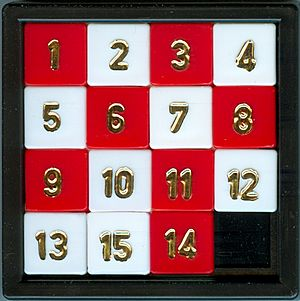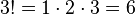# Permutation facts for kids

Kids Encyclopedia FactsIn the 15 puzzle the goal is to get the squares in ascending order. Initial positions which have an odd number of inversions are impossible to solve.

A permutation is a single way of arranging a group of objects. It is useful in mathematics.

A permutation can be changed into another permutation by simply switching two or more of the objects. For example, the way four people can sit in a car is a permutation. If some of them chose different seats, it would be a different permutation.

## Permutations without repetitions

The factorial has special application in defining the number of permutations in a set which does not include repetitions. The number n! is precisely the number of ways we can rearrange n things into a new order. For example, if we have three fruit: an orange, apple and pear, we can eat them in the order mentioned, or we can change them e.g. an apple, then a pear and finally an orange. The exact number of permutations is then$3! = 1 \cdot 2 \cdot 3 = 6$. The number gets extremely large as the number of items (n) goes up.Permutation Facts for Kids. Kiddle Encyclopedia.